# fillgaps

Fill gaps using autoregressive modeling

## Syntax

``y = fillgaps(x)``
``y = fillgaps(x,maxlen)``
``y = fillgaps(x,maxlen,order)``
``fillgaps(___)``

## Description

example

````y = fillgaps(x)` replaces any `NaN`s present in a signal `x` with estimates extrapolated from forward and reverse autoregressive fits of the remaining samples. If `x` is a matrix, then the function treats each column as an independent channel.```

example

````y = fillgaps(x,maxlen)` specifies the maximum number of samples to use in the estimation. Use this argument when your signal is not well characterized throughout its range by a single autoregressive process.```

example

````y = fillgaps(x,maxlen,order)` specifies the order of the autoregressive model used to reconstruct the gaps.```

example

````fillgaps(___)` with no output arguments plots the original samples and the reconstructed signal. This syntax accepts any input arguments from previous syntaxes.```

## Examples

collapse all

Load a speech signal sampled at ${F}_{s}=7418\phantom{\rule{0.2777777777777778em}{0ex}}Hz$. The file contains a recording of a female voice saying the word "MATLAB®." Play the sound.

```load mtlb % To hear, type soundsc(mtlb,Fs)```

Simulate a situation in which a noisy transmission channel corrupts parts of the signal irretrievably. Introduce gaps of random length roughly every 500 samples. Reset the random number generator for reproducible results.

```rng default gn = 3; mt = mtlb; gl = randi([300 600],gn,1); for kj = 1:gn mt(kj*1000+randi(100)+(1:gl(kj))) = NaN; end```

Plot the original and corrupted signals. Offset the corrupted signal for ease of display. Play the signal with the gaps.

```plot([mtlb mt+4]) legend('Original','Corrupted')```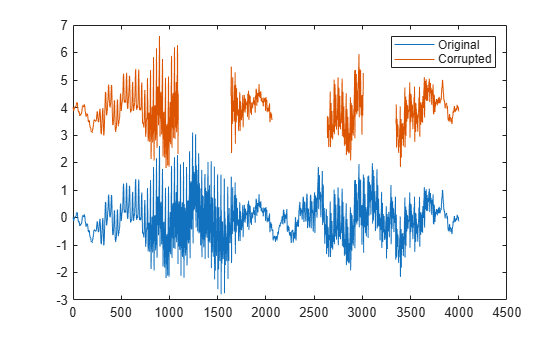`% To hear, type soundsc(mt,Fs)`

Reconstruct the signal using an autoregressive process. Use `fillgaps` with the default settings. Plot the original and reconstructed signals, again using an offset. Play the reconstructed signal.

```lb = fillgaps(mt); plot([mtlb lb+4]) legend('Original','Reconstructed')```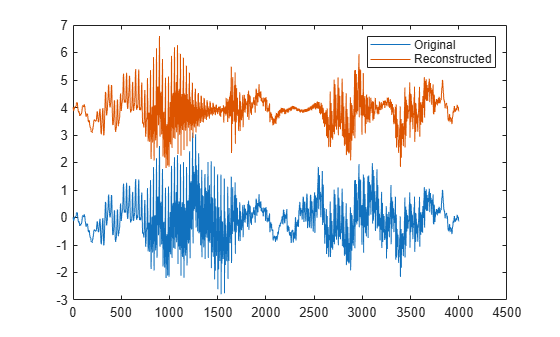`% To hear, type soundsc(lb,Fs)`

Load a file that contains depth measurements of a mold used to mint a United States penny. The data, taken at the National Institute of Standards and Technology, are sampled on a 128-by-128 grid.

`load penny`

Draw a contour plot with 25 copper-colored contour lines.

```nc = 25; contour(P,nc) colormap copper axis ij square```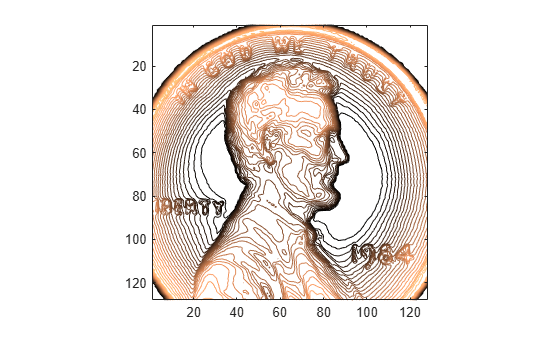Introduce four 10-by-10 gaps into the data. Draw a contour plot of the corrupted signal.

```P(50:60,80:90) = NaN; P(100:110,20:30) = NaN; P(100:110,100:110) = NaN; P(20:30,110:120) = NaN; contour(P,nc) colormap copper axis ij square```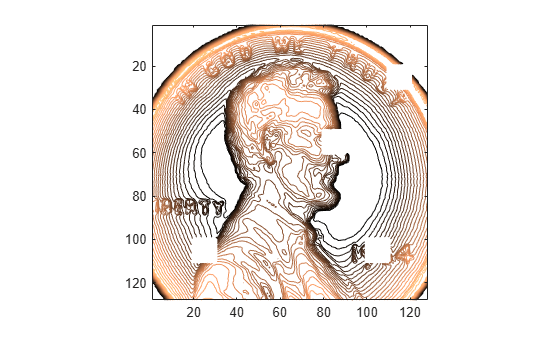Use `fillgaps` to reconstruct the data, treating each column as an independent channel. Specify an 8th-order autoregressive model extrapolated from 30 samples at each end. Draw a contour plot of the reconstruction.

```q = fillgaps(P,30,8); contour(q,nc) colormap copper axis ij square```Generate a function that consists of the sum of two sinusoids and a Lorentzian curve. The function is sampled at 200 Hz for 2 seconds. Plot the result.

```x = -1:0.005:1; f = 1./(1+10*x.^2)+sin(2*pi*3*x)/10+cos(25*pi*x)/10; plot(x,f)```Insert gaps at intervals (-0.8,-0.6), (-0.2,0.1), and (0.4,0.7).

```h = f; h(x>-0.8 & x<-0.6) = NaN; h(x>-0.2 & x< 0.1) = NaN; h(x> 0.4 & x< 0.7) = NaN;```

Fill the gaps using the default settings of `fillgaps`. Plot the original and reconstructed functions.

```y = fillgaps(h); plot(x,f,'.',x,y)```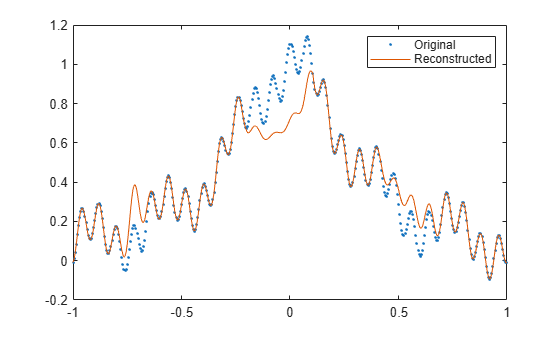Repeat the computation, but now specify a maximum prediction-sequence length of 3 samples and a model order of 1. Plot the original and reconstructed functions. At its simplest, `fillgaps` performs a linear fit.

```y = fillgaps(h,3,1); plot(x,f,'.',x,y)```Specify a maximum prediction-sequence length of 80 samples and a model order of 40. Plot the original and reconstructed functions.

```y = fillgaps(h,80,40); plot(x,f,'.',x,y)```Change the model order to 70. Plot the original and reconstructed functions.

```y = fillgaps(h,80,70); plot(x,f,'.',x,y)```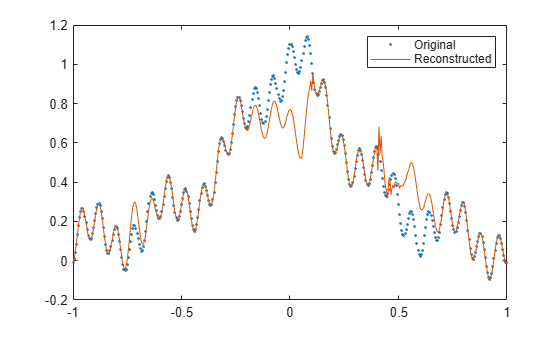The reconstruction is imperfect because very high model orders often have problems with finite precision.

Generate a multichannel signal consisting of two instances of a chirp sampled at 1 kHz for 1 second. The frequency of the chirp is zero at 0.3 seconds and increases linearly to reach a final value of 40 Hz. Each instance has a different DC value.

```Fs = 1000; t = 0:1/Fs:1-1/Fs; r = chirp(t-0.3,0,0.7,40); f = 1.1; q = [r-f;r+f]';```

Introduce gaps to the signal. One of the gaps covers the low-frequency region, and the other covers the high-frequency region.

```gap = (460:720); q(gap-300,1) = NaN; q(gap+200,2) = NaN;```

Fill the gaps using the default parameters. Plot the reconstructed signals.

```y = fillgaps(q); plot(t,y)```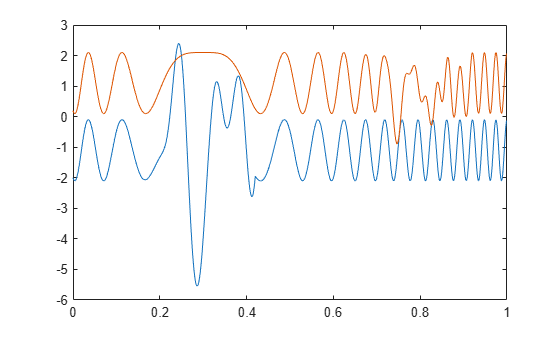Fill the gaps by fitting 14th-order autoregressive models to the signal. Limit the models to incorporate 15 samples on the end of each gap. Use the functionality of `fillgaps` to plot the reconstructions.

`fillgaps(q,15,14)`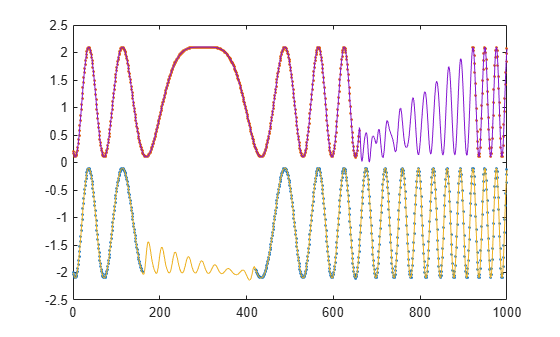Increase the number of samples to use in the estimation to 150. Increase the model order to 140.

`fillgaps(q,150,140)`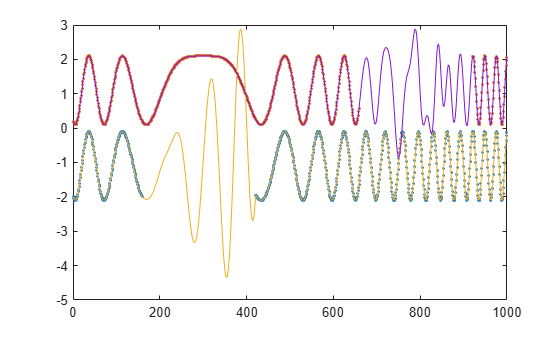## Input Arguments

collapse all

Input signal, specified as a vector or matrix. If `x` is a matrix, then its columns are treated as independent channels. `x` contains `NaN`s to represent missing samples.

Example: ```cos(pi/4*(0:159))+reshape(ones(32,1)*[0 NaN 0 NaN 0],1,160)``` is a single-channel row-vector signal missing 40% of its samples.

Example: ```cos(pi./[4;2]*(0:159))'+reshape(ones(64,1)*[0 NaN 0 NaN 0],160,2)``` is a two-channel signal with large gaps.

Data Types: `single` | `double`
Complex Number Support: Yes

Maximum length of prediction sequences, specified as a positive integer. If you leave `maxlen` unspecified, then `fillgaps` iteratively fits autoregressive models using all previous points for forward estimation and all future points for backward estimation.

Data Types: `single` | `double`

Autoregressive model order, specified as `'aic'` or a positive integer. The order is truncated when `order` is infinite or when there are not enough available samples. If you specify `order` as `'aic'`, or leave it unspecified, then `fillgaps` selects the order that minimizes the Akaike information criterion.

Data Types: `single` | `double` | `char` | `string`

## Output Arguments

collapse all

Reconstructed signal, returned as a vector or matrix.

 Akaike, Hirotugu. "Fitting Autoregressive Models for Prediction." Annals of the Institute of Statistical Mathematics. Vol. 21, 1969, pp. 243–247.

 Kay, Steven M. Modern Spectral Estimation: Theory and Application. Englewood Cliffs, NJ: Prentice Hall, 1988.

 Orfanidis, Sophocles J. Optimum Signal Processing: An Introduction. 2nd Edition. New York: McGraw-Hill, 1996.